Next: 3.2 Stress Up: 3. Strain and Semiconductor Previous: 3. Strain and Semiconductor

# 3.1 Strain

First a basic set of expressions to describe strain in semiconductors is introduced [161,162,163]. The orthonormal vector set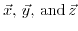is the basis of the unstrained solid.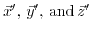represent the distorted vectors under a uniform deformation,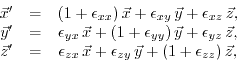(3.1)

where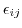are the deformation coefficients of the system.

Assuming a uniform deformation, a point located at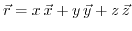will be shifted to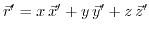, which leads to the displacement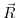defined as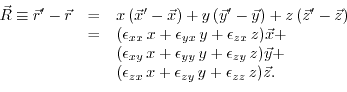(3.2)

Further generalization leads to a description for non-uniform deformation, introducing a position dependent vector function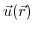,(3.3)

Restricting to small displacements from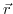, the displacement functioncan be developed into a Taylor series and truncated after the linear term at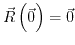, leading to a relation between the local displacement tensor and the displacement function,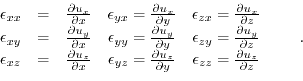Therefore, the displacement can be expressed as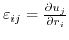. Frequently, the displacements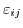are expressed via the strain tensorto describe the deformation of a body in three dimensions. In the limit of small deformations, the strain tensor is known as the Green tensor or Cauchy's infinitesimal strain tensor,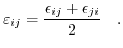(3.4)

The relative length change in the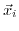direction is described by the diagonal coefficients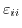, while the off-diagonal elements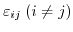denote the angular distortions by shear strains.

Also very common are the engineering strains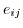, which are linked with the strain tensor as follows: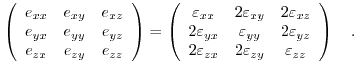(3.5)

The Voigt notation uses the six indipendent components of the strain tensor in a more compact vector form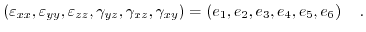(3.6)Next: 3.2 Stress Up: 3. Strain and Semiconductor Previous: 3. Strain and Semiconductor

T. Windbacher: Engineering Gate Stacks for Field-Effect Transistors